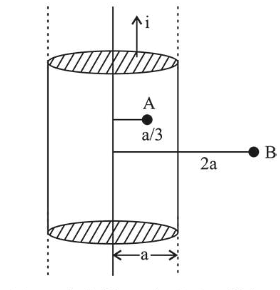# A long, straight wire of radius a carries a current distributed uniformly over its cross-section. The ratio of the magnetic

Question:

A long, straight wire of radius a carries a current distributed uniformly over its cross-section. The ratio of the magnetic fields due to the wire at distance $\frac{a}{3}$ and $2 a$, respectively from the axis of the wire is:

1. (1) $\frac{2}{3}$

2. (2) 2

3. (3) $\frac{1}{2}$

4. (4) $\frac{3}{2}$

Correct Option: 1

Solution:

(1) Let $a$ be the radius of the wire

Magnetic field at point $A$ (inside)

$B_{A}=\frac{\mu_{0} i r}{2 \pi a^{2}}=\frac{\mu_{0} i \frac{a}{3}}{2 \pi a^{2}}=\frac{\mu_{0} i}{\pi a^{2}} \frac{a}{6}=\frac{\mu_{0} i}{6 \pi a}$Magnetic field at point B (outside)

$B_{B}=\frac{\mu_{0} i}{2 \pi(2 a)}$

$\therefore \quad \frac{B_{A}}{B_{B}}=\frac{\frac{\mu_{0} i}{6 \pi a}}{\frac{\mu_{0} i}{2 \pi(2 \mathrm{a})}}=\frac{4}{6}=\frac{2}{3}$